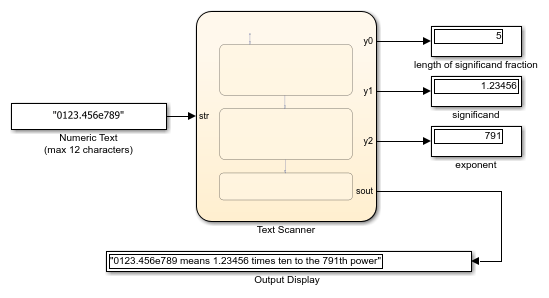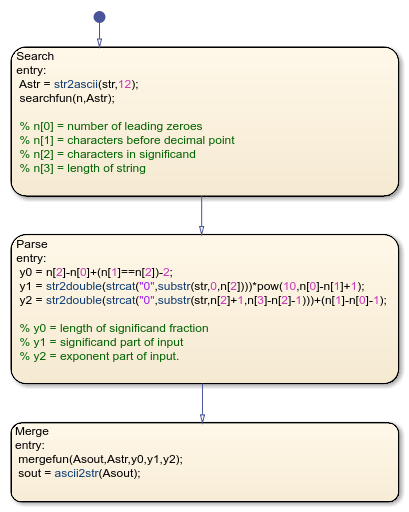# Share String Data with Custom C Code

This example shows how to share string data between a Stateflow® chart and custom C code. You can export string data from a Stateflow chart to a C function by using the `str2ascii` operator. You can import the output of your C code as string data in a Stateflow chart by using the `ascii2str` operator. By sharing data with custom code, you can augment the capabilities of Stateflow and leverage the software to take advantage of your preexisting code. For more information, see Reuse Custom Code in Stateflow Charts.This model contains a Stateflow chart that calls two functions from custom C code. During simulation, the chart takes as its input a string that contains text representing a floating-point number in exponential form. The chart consists of three states that:

• Search the input string for leading zeroes, a decimal point, and an `e`.

• Parse the string into double-precision numbers representing the significand and exponent parts of the input.

• Merge the numeric information into an output string expressing the input in scientific notation.

For example, if the input string is `"0123.456e789"`, then the chart outputs the string `"0123.456e789 means 1.23456 times ten to the 791th power`".### Export String Data from Stateflow to C

You can use the `str2ascii` operator to convert string data into an array that you can export from a Stateflow chart to a custom C code function.

1. In the custom code function, declare the input variable as having type `char*`.

2. In the Stateflow chart, convert the string to an array of type `uint8` by calling the operator `str2ascii`.

3. Call the custom code function by passing the `uint8` array as an input.

For example, in the previous chart, the `Search` state converts the input string `str` to the `uint8` array `Asrt`. The `Search` state passes this array as an input to the custom code function `searchfun`:

```extern void searchfun(int* nout, char* strin) { nout = strspn(strin,"0"); nout = strcspn(strin,".e"); nout = strcspn(strin,"e"); nout = strlen(strin); }```

The `Search` state calls this function with the command `searchfun(n,Astr)`. The function populates the integer array `n` with these values:

• `n` contains the number of leading zeroes in the input string `str`.

• `n` contains the number of characters before the first instance of a decimal point or `e`. This result provides the number of characters before the decimal point in `str`.

• `n` contains the number of characters before the first instance of `e`. This result provides the number of characters in the significand in `str`.

• `n` contains the length of the input string `str`.

The `Parse` state uses these results to extract the values of the significand and exponent parts of the input.

### Import String Data from C to Stateflow

You can import string data to a Stateflow chart by passing a pointer to an array of type `uint8` as an input to a custom C function.

1. In the custom code function, declare the input variable containing the pointer as having type `char*`.

2. Save the output string data from the custom code function at the location indicated by the pointer.

3. In the Stateflow chart, convert the `uint8` array to a string by calling the operator `ascii2str`.

For example, in the previous chart, the `Merge` state consolidates the numeric information obtained by the `Parse` state into an output string by calling the custom code function `mergefun`:

```extern void mergefun(char* strout, char* strin, int in0, double in1, double in2) { sprintf(strout, "%s means %1.*f times ten to the %dth power", strin, in0, in1, (int) in2); }```

The `Merge` state calls the `mergefun` function with the command `mergefun(Asout,Astr,y0,y1,y2)`:

• `Asout` is an array of type `uint8` pointing to the output of the custom function.

• `Astr` is an array of type `uint8` corresponding to the input string to the chart.

• `y0` is an integer containing the number of digits to the right of the decimal point in the significand.

• `y1` and `y2` are double-precision numbers representing the significand and exponent parts of the input.

The function `mergefun` calls the C library function `sprintf`, merging the contents of `Astr`, `y1`, and `y2` and storing the result in the memory location indicated by `Aout`. The chart uses the operator `ascii2str` to convert this output to the string `sout`. In this way, the model imports the string constructed by the custom code function back into Stateflow.